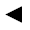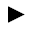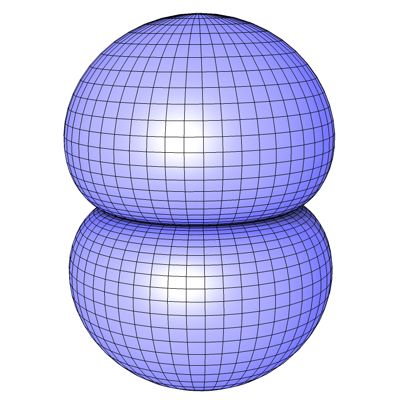[back]

## 3.38. Kidney Surface

[next]The kidney surface [ 8 ] is represented by the following equations.

 x = cos(u) (3 cos(v) - cos(3v)) 3-129 y = sin(u) (3 cos(v) - cos(3 v)) 3-130 z = 3sin(v) - sin(3v) 3-131

To represent the area, the two parameters u and v can assume the following values ​​(domain of definition), for example.

 u is an element from the set of numbers [0, 2 pi] v is an element from the number set [-pi/2, pi/2]

Since the Kidney Surface is a closed figure, the definition range must be adhered to exactly, it cannot be changed with the plugin.
The plugin creates an optimized mesh without duplicate points and unconnected polygons.Fig. 42